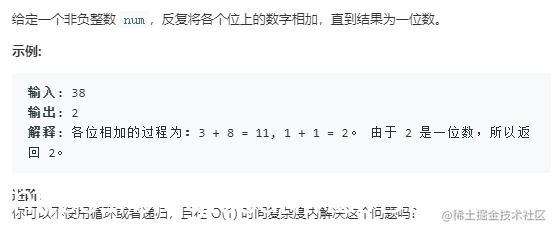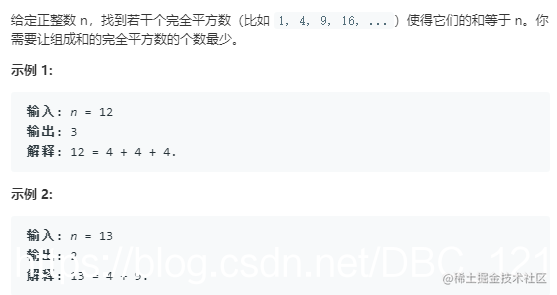# 一些通过数学分析解决的算法题汇总

## “树根”相关问题

“树根”在维基百科的定义如下：

``````原数: 1 2 3 4 5 6 7 8 9 10 11 12 13 14 15 16 17 18 19 20 21 22 23 24 25 26 27 28 29 30

• n 是 0 ，数根就是 0。
• n 不是 9 的倍数，数根就是 n 对 9 取余，即 n mod 9。
• n 是 9 的倍数，数根就是 9。

``````原数: 1 2 3 4 5 6 7 8 9 10 11 12 13 14 15 16 17 18 19 20 21 22 23 24 25 26 27 28 29 30``````public int addDigits(int num) {
return (num - 1) % 9 + 1;
}

## 四平方和定理

Legendre’s three-square theorem ，这个定理表明，如果正整数 n 被表示为三个平方数的和，那么 n n n 不等于 4 a ∗ ( 8 b + 7 ) 4^a*(8b+7) 4a∗(8b+7)， a a a 和 b b b 都是非负整数。换言之，如果 n = = 4 a ∗ ( 8 b + 7 ) n == 4^a*(8b+7) n==4a∗(8b+7) ，那么他一定不能表示为三个平方数的和，同时也说明不能表示为一个、两个平方数的和，因为如果能表示为两个平方数的和，那么补个 0 0 0，就能凑成三个平方数的和了。

• 首先考虑答案是不是 1 1 1，也就是判断当前数是不是一个平方数。
• 然后考虑答案是不是 4 4 4，也就是判断 n 是不是等于 4 a ∗ ( 8 b + 7 ) 4^a*(8b+7) 4a∗(8b+7)。
• 然后考虑答案是不是 2 2 2，当前数依次减去一个平方数，判断得到的差是不是平方数。
• 以上情况都排除的话，答案就是 3 3 3。``````public int numSquares(int n) {
if (isSquare(n)) {
return 1;
}

int temp = n;
while (temp % 4 == 0) {
temp /= 4;
}
if (temp % 8 == 7) {
return 4;
}

for (int i = 1; i * i < n; i++) {
if (isSquare(n - i * i)) {
return 2;
}
}

return 3;
}

private boolean isSquare(int n) {
int sqrt = (int) Math.sqrt(n);
return sqrt * sqrt == n;
}